Inverse of matrix using elementary transformation

Chapter 3 Class 12 Matrices (Term 1)
Concept wise

We have learned about elementary operations

Let’s learn how to find inverse of a matrix using it.

We will find inverse of a 2 × 2 & a 3 × 3 matrix

Note:- While doing elementary operations, we use

Only rows

OR

Only columns

Not both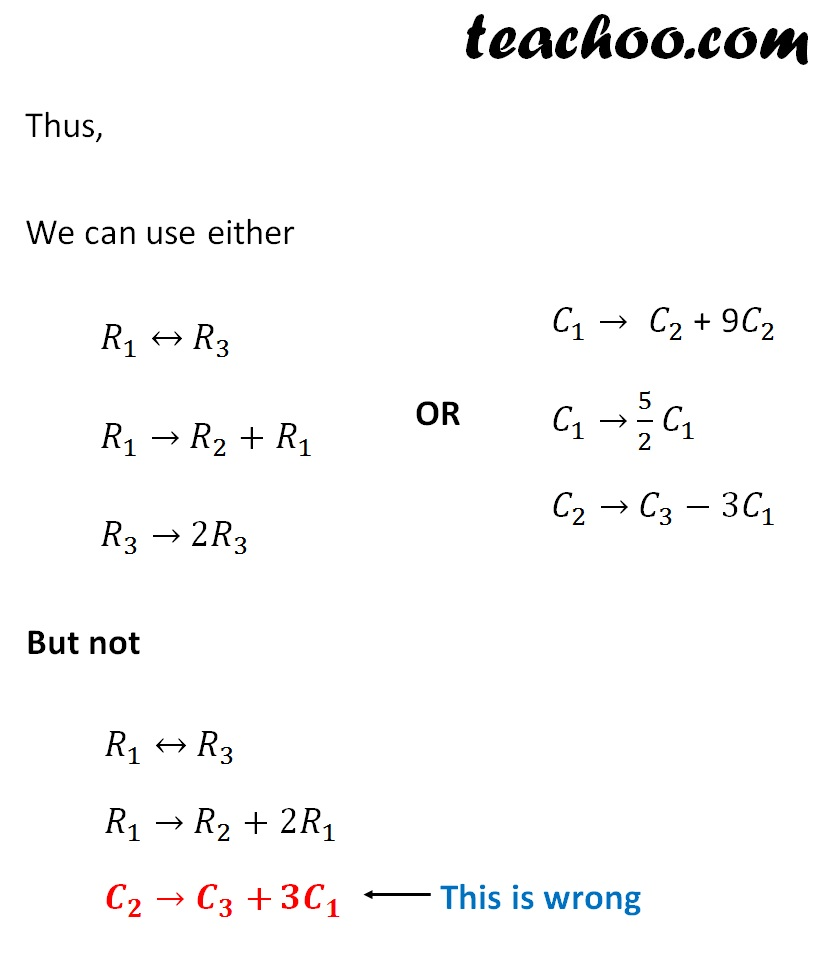Let's take some examples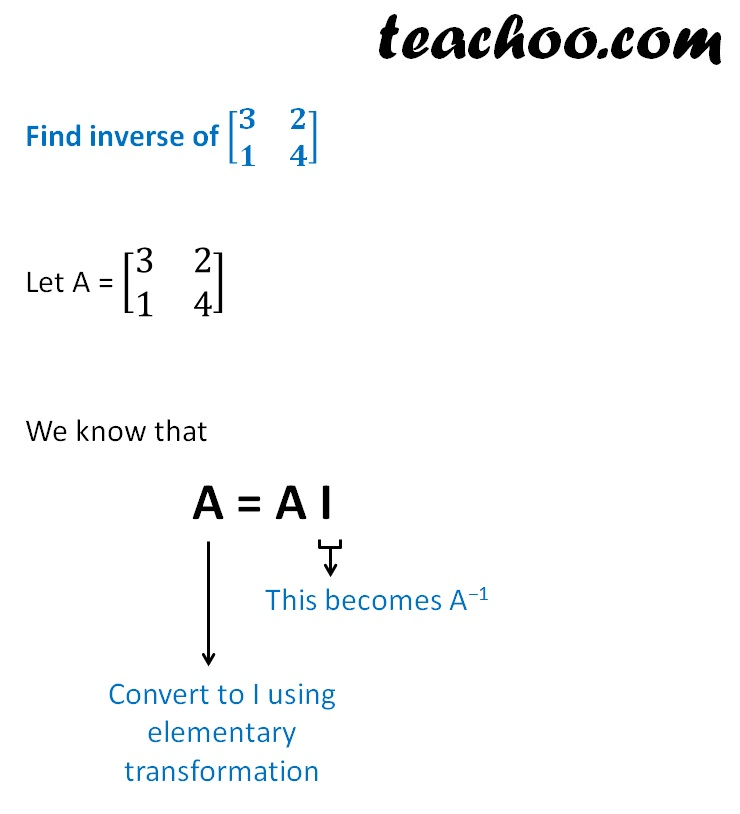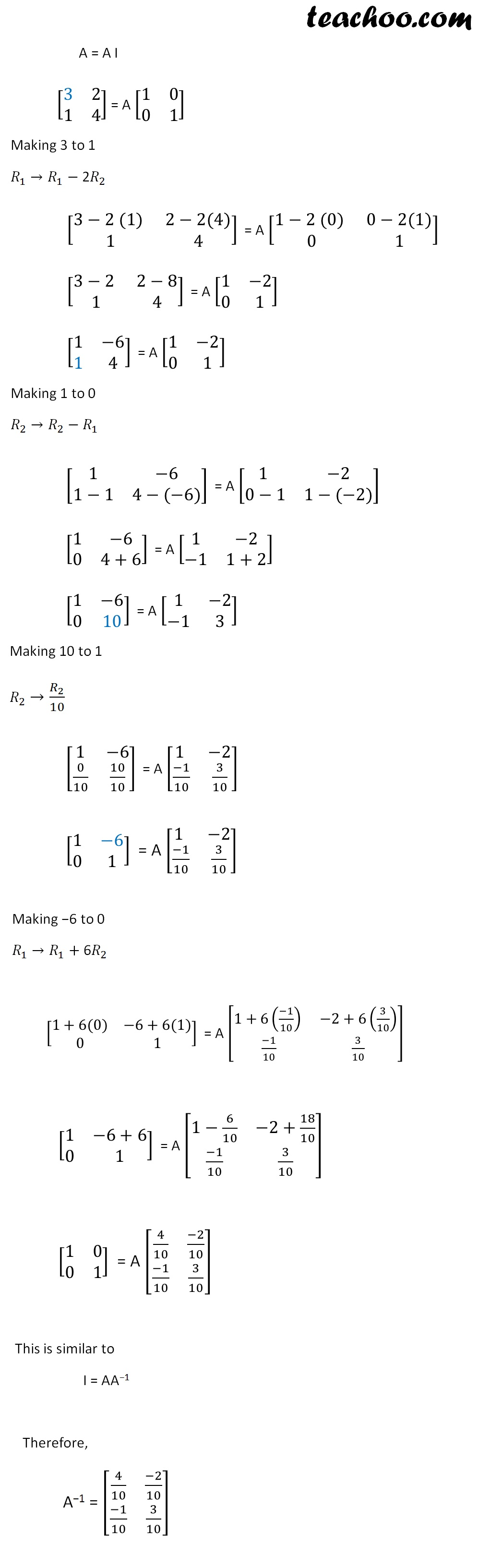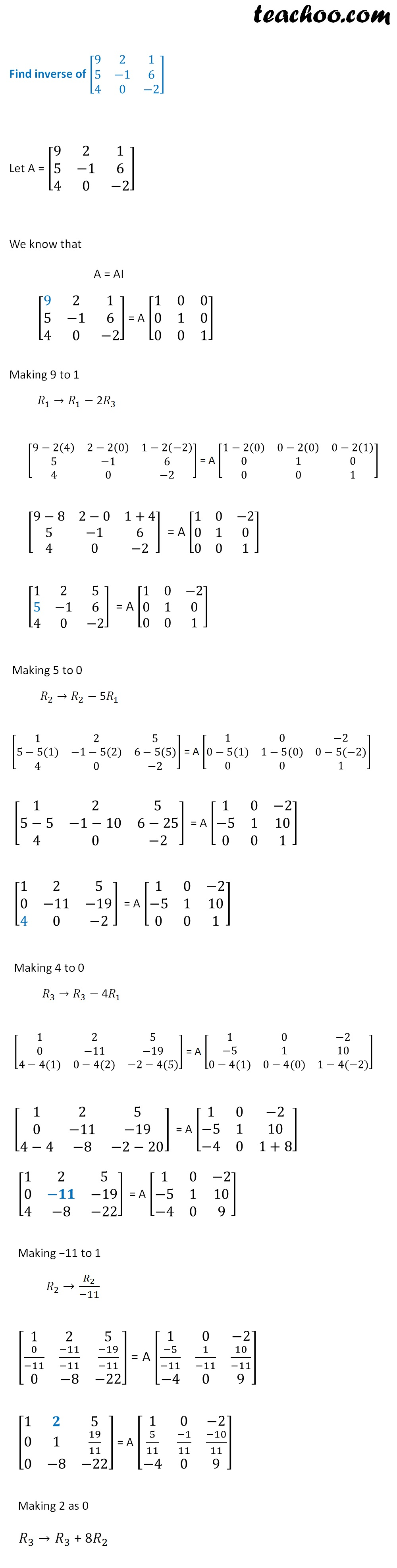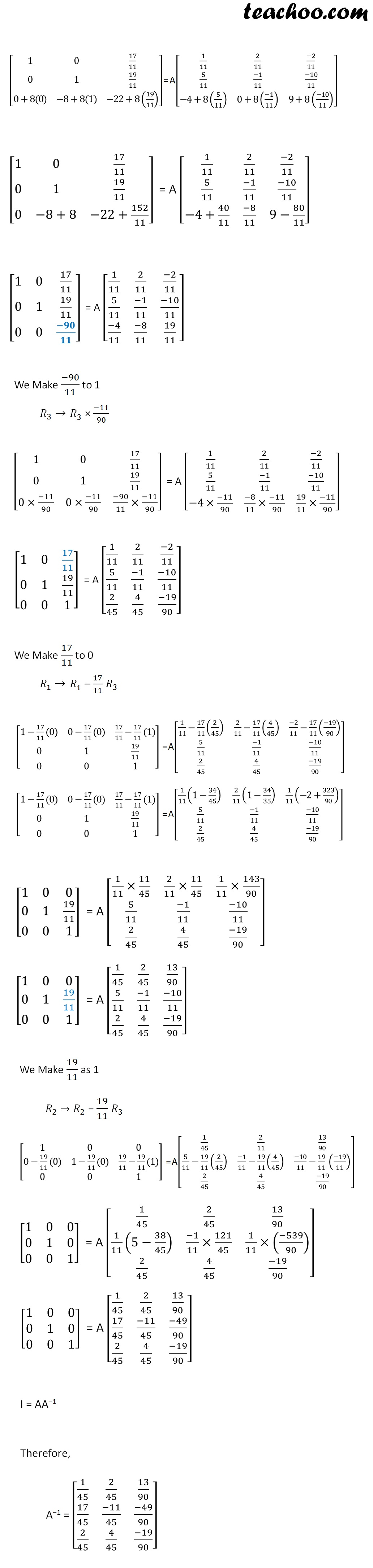### Transcript

Thus, We can use either 𝑅_1↔ 𝑅_3 𝑅_1→ 𝑅_2 + 𝑅_1 𝑅_3→ 2𝑅_3 OR 𝐶_1→" " 𝐶_2 + 9𝐶_2 𝐶_1→ 5/2 𝐶_1 𝐶_2→ 𝐶_3−3𝐶_1 But not 𝑅_1↔ 𝑅_3 𝑅_1→ 𝑅_2 + 〖2𝑅〗_1 𝑪_𝟐→ 𝑪_𝟑 + 〖𝟑𝑪〗_𝟏 This is wrong Find inverse of [■8(𝟑&𝟐@𝟏&𝟒)] Let A = [■8(3&2@1&4)] We know that A = A I This becomes A−1 Convert to I using elementary transformation A = A I [■8(3&2@1&4)] = A [■8(1&0@0&1)] Making 3 to 1 𝑅_1 →𝑅_1− 2𝑅_2 [■8(3−2 (1) &2−2(4)@1&4)] = A [■8(1−2 (0) &0−2(1)@0&1)] [■8(3−2 &2−8@1&4)] = A [■8(1&−2@0&1)] [■8(1&−6@1&4)] = A [■8(1&−2@0&1)] Making 1 to 0 𝑅_2 →𝑅_2− 𝑅_1 [■8(1&−6@1−1&4−(−6))] = A [■8(1 &−2@0−1&1−(−2))] [■8(1&−6@0&4+6)] = A [■8(1&−2@−1&1+2)] [■8(1&−6@0&10)] = A [■8(1&−2@−1&3)] Making 10 to 1 𝑅_2 →𝑅_2/10 [■8(1&−6@0/10&10/10)] = A [■8(1 &−2@(−1)/10&3/10)] [■8(1&−6@0&1)] = A [■8(1 &−2@(−1)/10&3/10)] Making −6 to 0 𝑅_1 →𝑅_1+ 6𝑅_2 [■8(1+6(0)&−6+6(1)@0&1)] = A [■8(1+6((−1)/10) &−2+6(3/10)@(−1)/10&3/10)] [■8(1&−6+6@0&1)] = A [■8(1−6/10&−2+18/10@(−1)/10&3/10)] [■8(1&0@0&1)] = A [■8(4/10&(−2)/10@(−1)/10&3/10)] This is similar to I = AA−1 Therefore, A−1 = [■8(4/10&(−2)/10@(−1)/10&3/10)] Find inverse of [■8(9&2&1@5&−1&6@4&0&−2)] Let A = [■8(9&2&1@5&−1&6@4&0&−2)] We know that A = AI [■8(9&2&1@5&−1&6@4&0&−2)] = A [■8(1&0&0@0&1&0@0&0&1)] Making 9 to 1 𝑅_1 →𝑅_1− 2𝑅_3 [■8(9−2(4)&2−2(0)&1−2(−2)@5&−1&6@4&0&−2)] = A [■8(1−2(0)&0−2(0)&0−2(1)@0&1&0@0&0&1)] [■8(9−8&2−0&1+4@5&−1&6@4&0&−2)] = A [■8(1&0&−2@0&1&0@0&0&1)] [■8(1&2&5@5&−1&6@4&0&−2)] = A [■8(1&0&−2@0&1&0@0&0&1)] Making 5 to 0 𝑅_2 →𝑅_2− 5𝑅_1 [■8(1&2&5@5−5(1)&−1−5(2)&6−5(5)@4&0&−2)] = A [■8(1&0&−2@0−5(1)&1−5(0)&0−5(−2)@0&0&1)] [■8(1&2&5@5−5&−1−10&6−25@4&0&−2)] = A [■8(1&0&−2@−5&1&10@0&0&1)] [■8(1&2&5@0&−11&−19@4&0&−2)] = A [■8(1&0&−2@−5&1&10@0&0&1)] Making 4 to 0 𝑅_3 →𝑅_3− 4𝑅_1 [■8(1&2&5@0&−11&−19@4−4(1)&0−4(2)&−2−4(5))] = A [■8(1&0&−2@−5&1&10@0−4(1)&0−4(0)&1−4(−2))] [■8(1&2&5@0&−11&−19@4−4&−8&−2−20)] = A [■8(1&0&−2@−5&1&10@−4&0&1+8)] [■8(1&2&5@0&−𝟏𝟏&−19@4&−8&−22)] = A [■8(1&0&−2@−5&1&10@−4&0&9)] Making −11 to 1 𝑅_2 →𝑅_2/(−11) [■8(1&2&5@0/(−11)&(−11)/(−11)&(−19)/(−11)@0&−8&−22)] = A [■8(1&0&−2@(−5)/(−11)&1/(−11)&10/(−11)@−4&0&9)] [■8(1&𝟐&5@0&1&19/11@0&−8&−22)] = A [■8(1&0&−2@5/11&(−1)/11&(−10)/11@−4&0&9)] Making 2 as 0 𝑅_1 →𝑅_1− 2𝑅_2 [■8(1−2(0)&2−2(1)&5−2(19/11)@0&1&19/11@0&−8&−22)] = A [■8(1−2(5/11)&0−2((−1)/11)&−2−2((−10)/11)@5/11&(−1)/11&(−10)/11@−4&0&9)] [■8(1&0&5−38/11@0&1&19/11@0&−8&−22)] = A [■8(1−10/11&2/11&−2+20/11@5/11&(−1)/11&(−10)/11@−4&0&9)] [■8(1&0&17/11@0&1&19/11@0&−𝟖&−22)] = A [■8(1/11&2/11&(−2)/11@5/11&(−1)/11&(−10)/11@−4&0&9)] Making −8 as 0 𝑅_3 →𝑅_3 + 8𝑅_2 [■8(1&0&17/11@0&1&19/11@0+8(0)&−8+8(1)&−22+8(19/11) )] = A [■8(1/11&2/11&(−2)/11@5/11&(−1)/11&(−10)/11@−4+8(5/11)&0+8((−1)/11)&9+8((−10)/11) )] [■8(1&0&17/11@0&1&19/11@0&−8+8&−22+152/11)] = A [■8(1/11&2/11&(−2)/11@5/11&(−1)/11&(−10)/11@−4+40/11&(−8)/11&9−80/11)] [■8(1&0&17/11@0&1&19/11@0&0&(−𝟗𝟎)/𝟏𝟏)] = A [■8(1/11&2/11&(−2)/11@5/11&(−1)/11&(−10)/11@(−4)/11&(−8)/11&19/11)] We Make (−90)/11 to 1 𝑅_3 →𝑅_3 × (−11)/90 [■8(1&0&17/11@0&1&19/11@0×(−11)/90&0×(−11)/90&(−90)/11×(−11)/90)] = A [■8(1/11&2/11&(−2)/11@5/11&(−1)/11&(−10)/11@−4×(−11)/90&(−8)/11×(−11)/90&19/11×(−11)/90)] [■8(1&0&17/11@0&1&19/11@0&0&1)] = A [■8(1/11&2/11&(−2)/11@5/11&(−1)/11&(−10)/11@2/45&4/45&(−19)/90)] We Make 17/11 to 0 𝑅_1 →𝑅_1 – 17/11 𝑅_3 [■8(1−17/11(0)&0−17/11(0)&17/11−17/11(1)@0&1&19/11@0&0&1)] = A [■8(1/11−17/11 (2/45)&2/11−17/11 (4/45)&(−2)/11−17/11 ((−19)/90)@5/11&(−1)/11&(−10)/11@2/45&4/45&(−19)/90)] [■8(1−17/11(0)&0−17/11(0)&17/11−17/11(1)@0&1&19/11@0&0&1)] = A [■8(1/11 (1−34/45) &2/11 (1−34/35)&1/11 (−2+323/90)@5/11&(−1)/11&(−10)/11@2/45&4/45&(−19)/90)] [■8(1&0&0@0&1&19/11@0&0&1)] = A [■8(1/11×11/45&2/11×11/45&1/11×143/90@5/11&(−1)/11&(−10)/11@2/45&4/45&(−19)/90)] [■8(1&0&0@0&1&19/11@0&0&1)] = A [■8(1/45&2/45&13/90@5/11&(−1)/11&(−10)/11@2/45&4/45&(−19)/90)] We Make 19/11 as 1 𝑅_2 →𝑅_2 − 19/11 𝑅_3 [■8(1&0&0@0−19/11(0)&1−19/11(0)&19/11−19/11(1)@0&0&1)] = A [■8(1/45&2/11&13/90@5/11−19/11 (2/45)&(−1)/11−19/11 (4/45)&(−10)/11−19/11 ((−19)/11)@2/45&4/45&(−19)/90)] [■8(1&0&0@0&1&0@0&0&1)] = A [■8(1/45&2/45&13/90@1/11 (5−38/45)&(−1)/11×121/45&1/11×((−539)/90)@2/45&4/45&(−19)/90)] [■8(1&0&0@0&1&0@0&0&1)] = A [■8(1/45&2/45&13/90@17/45&(−11)/45&(−49)/90@2/45&4/45&(−19)/90)] I = AA−1 Therefore, A−1 = [■8(1/45&2/45&13/90@17/45&(−11)/45&(−49)/90@2/45&4/45&(−19)/90)]